最优化算法之粒子群算法（PSO）

22 篇文章 68 订阅

粒子群优化算法(PSO：Particle swarm optimization) 是一种进化计算技术（evolutionary computation）。源于对鸟群捕食的行为研究。粒子群优化算法的基本思想：是通过群体中个体之间的协作和信息共享来寻找最优解．
PSO的优势：在于简单容易实现并且没有许多参数的调节。目前已被广泛应用于函数优化、神经网络训练、模糊系统控制以及其他遗传算法的应用领域。

1、基本思想

粒子群算法通过设计一种无质量的粒子来模拟鸟群中的鸟，粒子仅具有两个属性：速度和位置，速度代表移动的快慢，位置代表移动的方向。每个粒子在搜索空间中单独的搜寻最优解，并将其记为当前个体极值，并将个体极值与整个粒子群里的其他粒子共享，找到最优的那个个体极值作为整个粒子群的当前全局最优解，粒子群中的所有粒子根据自己找到的当前个体极值和整个粒子群共享的当前全局最优解来调整自己的速度和位置。下面的动图很形象地展示了PSO算法的过程：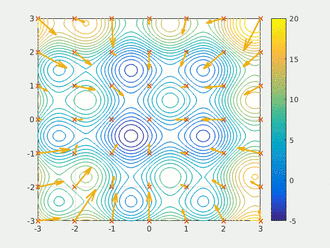2、更新规则

PSO初始化为一群随机粒子(随机解)。然后通过迭代找到最优解。在每一次的迭代中，粒子通过跟踪两个“极值”(pbest，gbest)来更新自己。在找到这两个最优值后，粒子通过下面的公式来更新自己的速度和位置。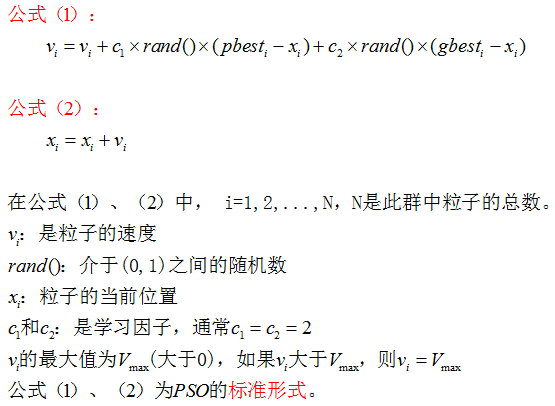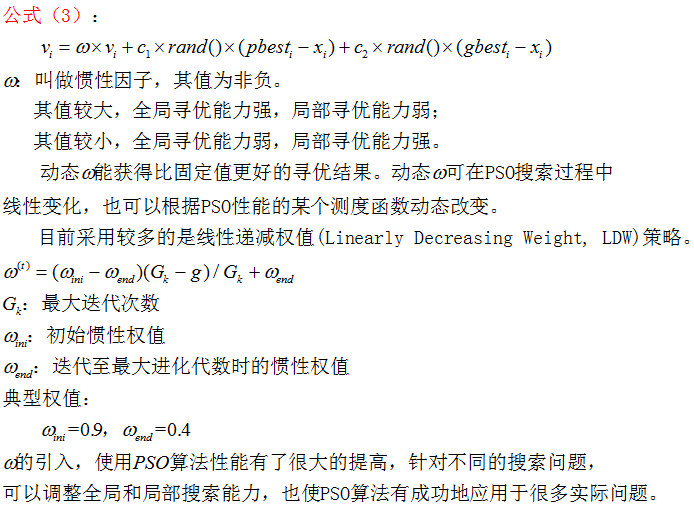3、PSO算法的流程和伪代码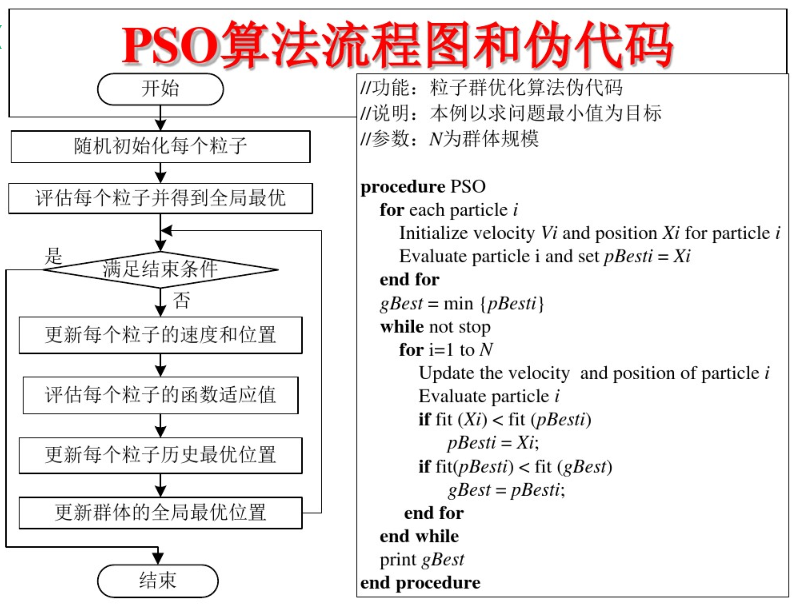4、PSO算法举例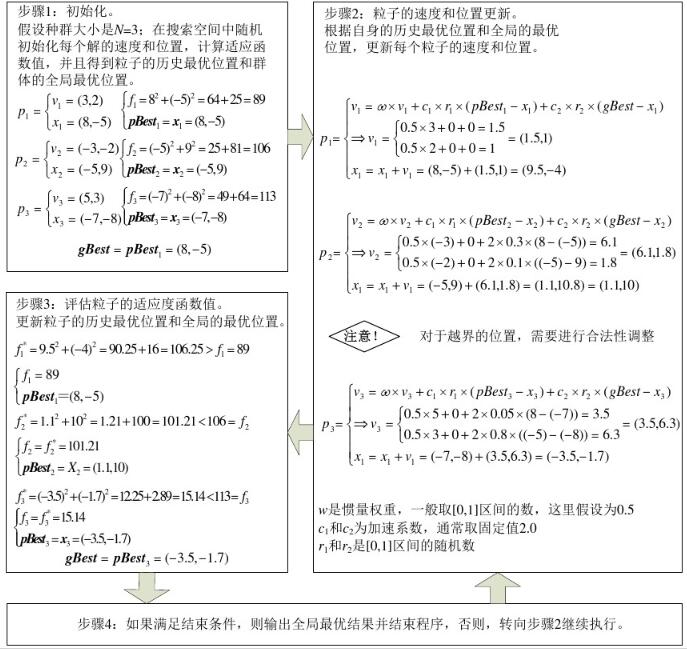5、PSO算法的demo

#include <iostream>
#include <vector>
#include <cmath>
#include <map>
#include <algorithm>
#include <random>
#include <ctime>
#include <Eigen/Dense>
using namespace Eigen;
using namespace std;

const int dim = 1;//维数
const int p_num = 10;//粒子数量
const int iters = 100;//迭代次数
const int inf = 999999;//极大值
const double pi = 3.1415;
//定义粒子的位置和速度的范围
const double v_max = 4;
const double v_min = -2;
const double pos_max = 2;
const double pos_min = -1;
//定义位置向量和速度向量
vector<double> pos;
vector<double> spd;
//定义粒子的历史最优位置和全局最优位置
vector<double> p_best;
double g_best;
//使用eigen库定义函数值矩阵和位置矩阵
Matrix<double, iters, p_num> f_test;
Matrix<double, iters, p_num> pos_mat;

//定义适应度函数
double fun_test(double x)
{
double res = x * x + 1;
return res;
}

//初始化粒子群的位置和速度
void init()
{
//矩阵中所有元素初始化为极大值
f_test.fill(inf);
pos_mat.fill(inf);
//生成范围随机数
static std::mt19937 rng;
static std::uniform_real_distribution<double> distribution1(-1, 2);
static std::uniform_real_distribution<double> distribution2(-2, 4);
for (int i = 0; i < p_num; ++i)
{
pos.push_back(distribution1(rng));
spd.push_back(distribution2(rng));
}
vector<double> vec;
for (int i = 0; i < p_num; ++i)
{
auto temp = fun_test(pos[i]);//计算函数值
//初始化函数值矩阵和位置矩阵
f_test(0, i) = temp;
pos_mat(0, i) = pos[i];
p_best.push_back(pos[i]);//初始化粒子的历史最优位置
}
std::ptrdiff_t minRow, minCol;
f_test.row(0).minCoeff(&minRow, &minCol);//返回函数值矩阵第一行中极小值对应的位置
g_best = pos_mat(minRow, minCol);//初始化全局最优位置
}

void PSO()
{
static std::mt19937 rng;
static std::uniform_real_distribution<double> distribution(0, 1);
for (int step = 1; step < iters; ++step)
{
for (int i = 0; i < p_num; ++i)
{
//更新速度向量和位置向量
spd[i] = 0.5 * spd[i] + 2 * distribution(rng) * (p_best[i] - pos[i]) +
2 * distribution(rng) * (g_best - pos[i]);
pos[i] = pos[i] + spd[i];
//如果越界则取边界值
if (spd[i] < -2 || spd[i] > 4)
spd[i] = 4;
if (pos[i] < -1 || pos[i] > 2)
pos[i] = -1;
//更新位置矩阵
pos_mat(step, i) = pos[i];
}
//更新函数值矩阵
for (int i = 0; i < p_num; ++i)
{
auto temp = fun_test(pos[i]);
f_test(step, i) = temp;
}
for (int i = 0; i < p_num; ++i)
{
MatrixXd temp_test;
temp_test = f_test.col(i);//取函数值矩阵的每一列
std::ptrdiff_t minRow, minCol;
temp_test.minCoeff(&minRow, &minCol);//获取每一列的极小值对应的位置
p_best[i] = pos_mat(minRow, i);//获取每一列的极小值，即每个粒子的历史最优位置
}
g_best = *min_element(p_best.begin(), p_best.end());//获取全局最优位置
}
cout << fun_test(g_best);
}

int main()
{
init();
PSO();
system("pause");
return 0;
}
12-13

05-05
06-0429万+
05-053094
11-061万+
06-1110万+
02-011万+
08-224531
07-0414万+
09-30
11-238875
06-222万+
06-04497
03-031万+
03-313万+
04-1812万+
10-17321lx青萍之末

¥2 ¥4 ¥6 ¥10 ¥20余额支付 (余额：-- )扫码支付获取中扫码支付点击重新获取扫码支付1.余额是钱包充值的虚拟货币，按照1:1的比例进行支付金额的抵扣。
2.余额无法直接购买下载，可以购买VIP、C币套餐、付费专栏及课程。余额充值